×#### Thank you for registering.

One of our academic counsellors will contact you within 1 working day.

Click to Chat

1800-1023-196

+91-120-4616500

CART 0

• 0

MY CART (5)

Use Coupon: CART20 and get 20% off on all online Study Material

ITEM
DETAILS
MRP
DISCOUNT
FINAL PRICE
Total Price: Rs.

There are no items in this cart.
Continue Shopping• Complete JEE Main/Advanced Course and Test Series
• OFFERED PRICE: Rs. 15,900
• View Details

```Chapter 13: Profits, Loss, Discount and Value Added Tax Exercise – 13.3

Question: 1

The list price of a refrigerator is Rs.9700. If a value-added tax of 6% is to be charged on it, how much one has to pay to buy the refrigerator?

Solution:

List price of the refrigerator = Rs. 9700

VAT = 6%

So, VAT = 6% of Rs.9700

= (6/100) × 9700

= Rs. 582

So, the total amount one has to pay is (Rs. 9700 + Rs.582) = Rs.10282

The total amount one has to pay is Rs. 10282.

Question: 2

Vikram bought a watch for Rs. 825. If this amount includes 10% VAT on the list price, what was the list price of the watch?

Solution:

Let the list price of the watch be Rs. x

Then, x + (10x/100) = 825x + 0.1x = 8251.1x = 825x = 8251.1x = Rs.750

Thus the list price of the watch is Rs.750.

Question: 3

Aman bought a shirt for Rs. 374.50 which includes 7 % VAT. Find the list price of the shirt.

Solution:

Let the list price of the shirt be Rs. x

Then,

= x + (7/100) = 374.50x + 0.07x = Rs.350

The list price of the shirt is Rs. 350.

Question: 4

Rani purchases a pair of shoes whose sale price is Rs.175. if she pays VAT at the same rate of 7%, how much amount does she pay as VAT? Also, find the net value of the pair of the pair of shoes.

Solution:

Given, S.P of the pair of shoes = Rs.175

VAT = 7%

Therefore, 7% of 175

= (7/100) × 175

= Rs. 12.25

Rani has to pay Rs.12.25 as VAT

Total cost = Rs. 175 + Rs.12.25 = Rs. 187.25

Question: 5

Swarna paid Rs. 20 as Vat on a pair of shoes worth Rs.250. find the rate of the VAT.

Solution:

Given, amount paid by Swarna for a pair of shoes = Rs.250

VAT paid by her = Rs. 20

Let the rate of VAT be x%

Then, x% of 250

= (x/100) × 250 = 8

= 2.5x = 8

= x = 8

Swarna paid 8% VAT on the pair of shoes.

Question: 6

Sarita buys goods worth Rs.5500. she gets a rebate of 5% on it. After getting the rebate if VAT at the rate of 5% is charged, find the amount she will have to pay for the goods.

Solution: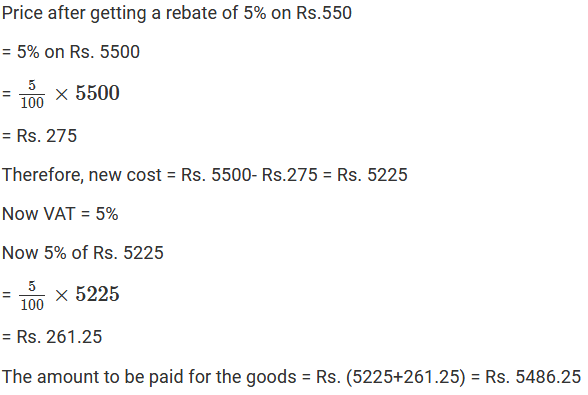Question: 7

The cost of furniture inclusive of the VAT is Rs. 7150. If the rate of the VAT is 105, find the original cost of the furniture.

Solution:

Cost of the furniture inclusive VAT = Rs. 7150

Let the original cost of the furniture be Rs. x

Cost of the furniture = 10% of x + x = Rs. 7150

= 1.10x = 7150

= x = Rs.6500

Thus, the original cost of the furniture is Rs. 6500.

Question: 8

A refrigerator is available for Rs. 13750 including VAT. If the rate of the VAT is 10%, find the original cost of the furniture.

Solution:

Cost of the refrigerator inclusive VAT is = Rs. 13750

Let the original cost of the furniture be Rs. x

Cost of the furniture = 10% of x + x = 13750

=1.10x = 13750

= x = Rs. 12500

Thus, the original cost of the furniture is Rs. 12500

Question: 9

A color TV is available for Rs. 134400 inclusive of VAT. If the original cost of the TV is Rs. 12000, find the rate of VAT.

Solution:

Cost price of the TV including VAT = Rs. 13440

Let the rate of VAT be x%

Cost of the TV = x% of 1200 + 1200

= 13440 - 12000 = 120x

= 120x = 1440

= x = 12

Thus, the rate of the VAT on the color TV is 12%

Question: 10

Reena goes to a shop to buy a radio, costing Rs. 2568. The rate of the VAT is 7%. She tells the shopkeeper to reduce the price of the radio such that she has to pay Rs. 2568, inclusive of VAT. Find the reduction needed at the price of radio.

Solution: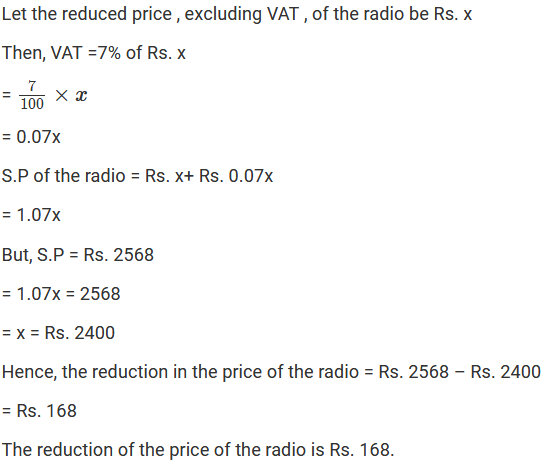Question: 11

Rajat goes to a departmental store and buys the following articles:

Item
Price per item
Rate of VAT

2 pairs of shoes
Rs.800
5%

1 sewing machine
Rs. 1500
6%

2 tea- sets
Rs. 650
4%

Calculate the total amount he has to pay to the store.

Solution:

Given, C.P of 2 pair of shoes = Rs. 800(2) = Rs.1600

Rate of VAT = 5%

= 5% of 1600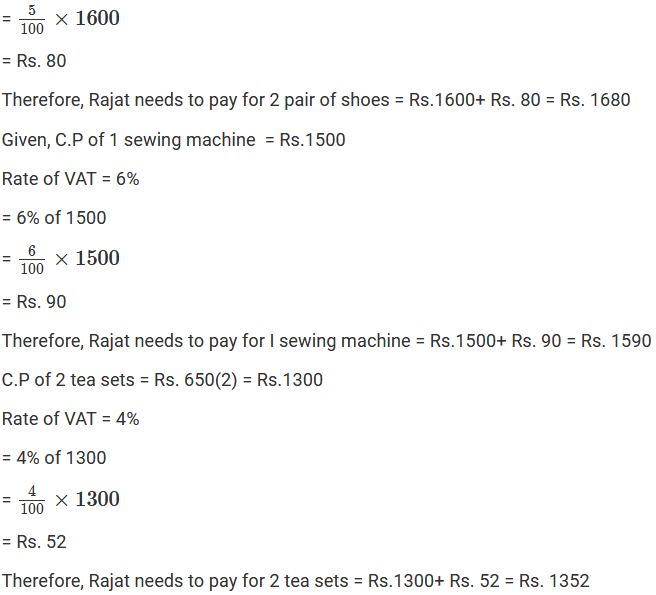Question: 12

Ajit buys a motorcycle for Rs. 17600 including value-added tax. If the rate of the VAT is 10%, what is the sale price of the motorcycle?

Solution:Question: 13

Manoj buys a leather coat costing Rs.900 and Rs.990 after paying VAT. Calculate the rate of VAT charged on the coat?

Solution:Question: 14

Rakesh goes to a departmental store and purchases the following articles:

(i) Biscuits and bakery products costing Rs. 50, VAT @ 5%

(ii) Medicines coating Rs.90, VAT @10%

(iii) Clothes costing Rs.400. VAT@1%

(iv) Cosmetics costing Rs.150, VAT@10%

Calculate the total amount to be paid by Rakesh to the store.

Solution: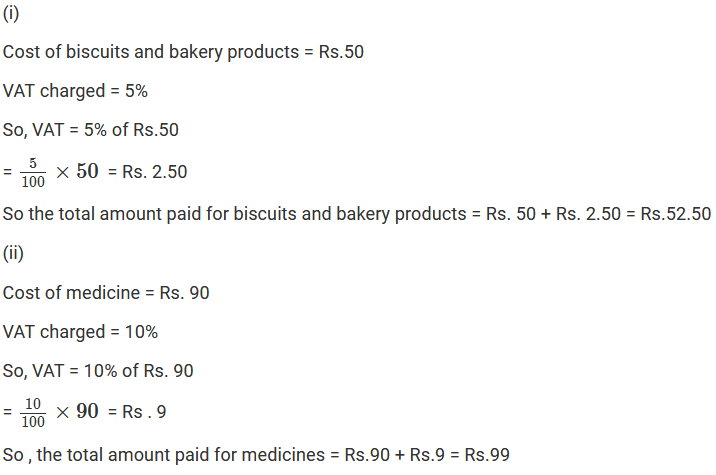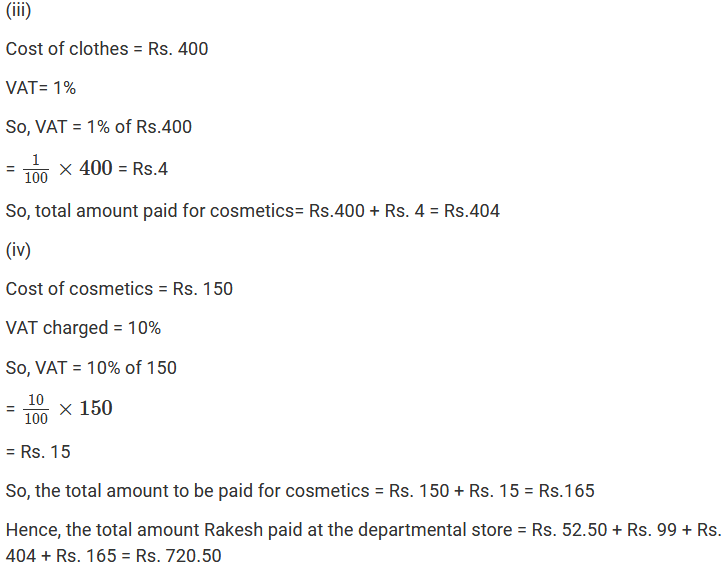Question: 15

Rajeeta purchased a set of cosmetics. She paid Rs.165 for it including VAT. If the rate of Vat is 10%, find the sale price of the set.

Solution:

Let the sale price of the set be Rs. x

Given that the VAT charged is 10%

Cost of the set = x + 10% of x = 165

= 1.10x = 165

= x = Rs.150

Thus, the sale price of the set is Rs. 150

Question: 16

Sunita purchases a bicycle for Rs.660. she has paid a VAT of 10%. Find the list price of the bicycle.

Solution:

Let the sale price of the bicycle be Rs. x

VAT charged = 10%

Again, cost of the bicycle = x + 10% of x = 660

= 1.10x = 660

= x = Rs.600

Thus, the list price of the bicycle is Rs. 600

Question: 17

The sales price of a television, inclusive of the VAT is Rs.13500. if the VAT is charged at the rate of 8 % of the list price, find the list price of the television.

Solution:

Let the list price be the TV be Rs. x

VAT charged = 8%

Given, cost price of the TV = Rs. 13500

So, cost price of the TV = x + 8% of x

= x+ 0.08x = 13500

= x = 12500

Thus the list price of the TV is Rs. 12500

Question: 18

Shikha purchased a car with marked price Rs.210000 at a discount of 5%. If the VAT is charged at the rate of 10%, find the amount she had paid for purchasing the car.

Solution: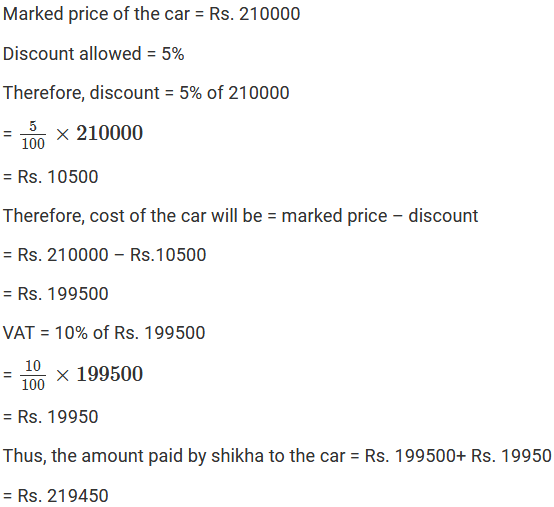Question: 19

Shruti bought a set of cosmetic items for Rs.345 including 15% value-added tax and a purse for Rs.110 including 10% VAT. What percent is the VAT charged on the whole transaction?

Solution: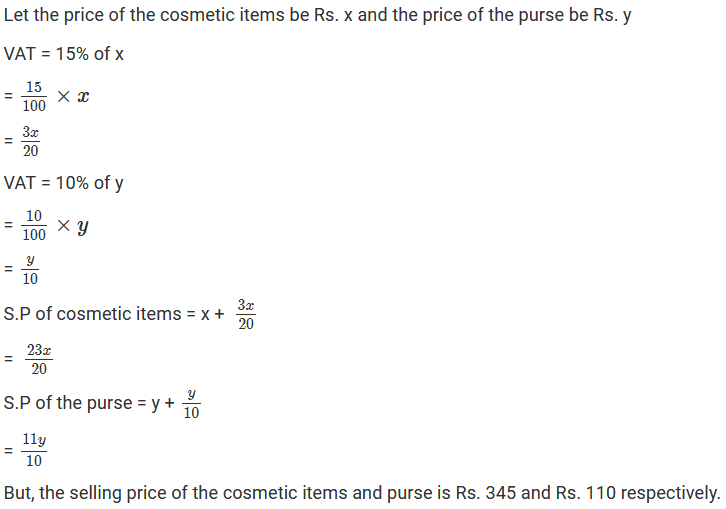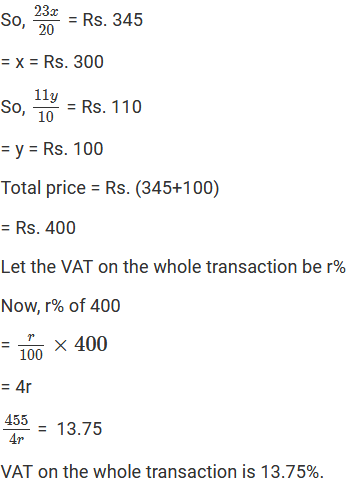Question: 20

The list price of the cooler is Rs. 2563. The rate of the VAT is 10%. The customer requests the shopkeeper to allow a discount in the price of the cooler to such an extent that the price remains Rs. 2563 inclusive of VAT. Find the discount in the price of the cooler.

Solution:Question: 21

The list price of a washing machine is Rs. 9000. If the dealer allows a discount of 5% on the cash payment, how much money will a customer pay to the dealer in cash, if the rate of the VAT is 10%?

Solution: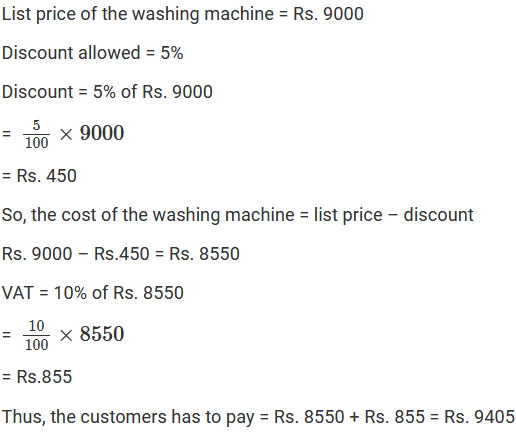```### Course Features

• 728 Video Lectures
• Revision Notes
• Previous Year Papers
• Mind Map
• Study Planner
• NCERT Solutions
• Discussion Forum
• Test paper with Video Solution